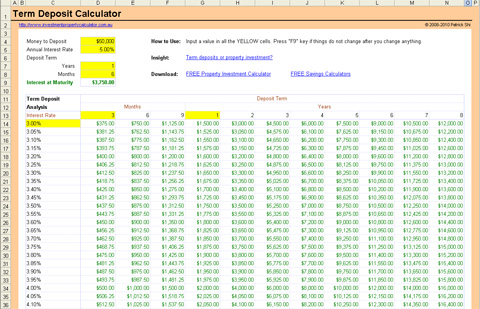Affiliate Program

ULTIMATE
Investment Property Calculator

[ v1.0.2019 ] - [ cost: \$149.99 ] - [ details ]

PROFESSIONAL
Investment Property Calculator

[ v1.0.2019 ] - [ cost: \$64.99 ] - [ details ]

STANDARD
Investment Property Calculator

[ v1.0.2019 ] - [ cost: \$44.99 ] - [ details ]

FREE
Investment Property Calculator

[ v1.0.2019 ] - [ cost: \$0.00 ] - [ details ]

\$190 PROPERTY
Depreciation Schedule Report

[ hot deal ] - [ cost: from \$190.00 ] - [ details ]

COMMERCIAL PROPERTY

[ v1.0.2019 ] - [ cost: \$299.99 ] - [ details ]

FREE Term Deposit Calculator

How much interest you can earn from bank term deposits?

This free term deposit calculator will show you how much interest you can get from a fixed term deposit.

In addition, you can use this calculator to compare bank term deposits with different term deposit interest rates and terms to see which is the best term deposit. For example, a Westpac 3 months 8% interest rate term deposit or an ANZ 1 year 6.8% interest rate term deposit.

This term deposit calculator also shows you how much interest the same amount money can earn under different term deposit interest rates and deposit terms, for example, 3, 6, 9 months or 1 to 8 years. You can change the interest rate, money to deposit, and how long you want to deposit with the banks.

Here is a screen shot that will give you a better idea that what you need to do and what this free Term Deposit Calculator can tell you.

This calculator is built in Microsoft Excel worksheet. You need to have Microsoft Excel 2000-2010 and Microsoft Windows to use it. You may be able to use the calculator with other applications that can open and read XLS spreadsheets, but this has not been tested.View full size image of FREE Term Deposit Calculator